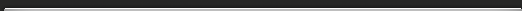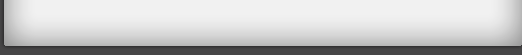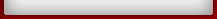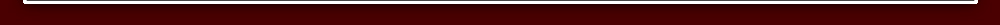Tutorial 4

## Tutorial 4

A quick way to square numbers that end in 5 using the formula BY ONE MORE THAN THE ONE BEFORE.

752 = 5625
75 means 75 x 75.
The answer is in two parts: 56 and 25.
The last part is always 25.
The first part is the first number, 7, multiplied by the number "one more", which is 8:
so 7 x 8 = 56Similarly 852 = 7225 because 8 x 9 = 72.

Exercise 1 Tutorial 4

Try these:
1) 452 =
2) 652 =
3) 952 =
4) 352 =
5) 152 =

Method for multiplying numbers where the first figures are the same and the last figures add up to 10.

32 x 38 = 1216

Both numbers here start with 3 and the last figures (2 andadd up to 10.
So we just multiply 3 by 4 (the next number up) to get 12 for the first part of the answer.
And we multiply the last figures: 2 x 8 = 16 to get the last part of the answer.
Diagrammatically:And 81 x 89 = 7209
We put 09 since we need two figures as in all the other examples.

Exercise 2 Tutorial 4

Practise some:

1) 43 x 47 =
2) 24 x 26 =
3) 62 x 68 =
4) 17 x 13 =
5) 59 x 51 =
6) 77 x 73 =